• 遗传线性回归：通过遗传算法进行线性回归拟合的近似
• 器学习一次回归和二次回归 reshape（行，列）可以根据指定的数值将...#采用线性回归方程预测 lr=LinearRegression() lr.fit (X,y) from sklearn.linear_model import LinearRegression lr = LinearRegression() # 训...
器学习一次回归和二次回归
reshape（行，列）可以根据指定的数值将数据转换为特定的行数和列数###reshape（-1，1）之后，数据集变成了一列
#采用线性回归方程预测
lr=LinearRegression()
lr.fit (X,y)
from sklearn.linear_model import LinearRegression
lr = LinearRegression()
# 训练模型(为了方便没有拆封训练集和测试集)
X = x.reshape(-1,1)
print(X.shape)
lr.fit(X,y)
# 训练过程就是寻找最佳权重和偏置的过程
print('权重',lr.coef_,'偏置',lr.intercept_)
y_predict = lr.predict(X)
plt.scatter(x,y)
# 生成线性图,观察预测值与真实值的误差
plt.plot(x,y_predict,color='r')
plt.show()
print(lr.score(X,y))
一次线性方程不合适，转变为二次线性方程
# y = w * x + b ===>  y = w1 * x**2 + w2 * x + b
print((X**2).shape)
# hstack 在水平方向追加, vstack垂直方向叠加
X2 = np.hstack([X**2,X])   # 将x^2 作为跟X一样的参数，使多计算一个权重
print(X2.shape)

##

python
import numpy as np
import matplotlib.pyplot as plt
# 在指定[-3,3]随机生成size个随机数(代表的特征值)
x = np.random.uniform(-3,3,size=100)
print(x,x.shape)
# 模拟目标值(y) 与x并不是简单的线性关系
y = 0.5 * x**2 + x + 2 + np.random.normal(0,1,size=100)

# 采用线性回归的方程来预测
from sklearn.linear_model import LinearRegression
lr = LinearRegression()
# 训练模型(为了方便没有拆封训练集和测试集)
X = x.reshape(-1,1)
print(X.shape)
lr.fit(X,y)
# 训练过程就是寻找最佳权重和偏置的过程
print('权重',lr.coef_,'偏置',lr.intercept_)
y_predict = lr.predict(X)
plt.scatter(x,y)
# 生成线性图,观察预测值与真实值的误差
plt.plot(x,y_predict,color='r')
plt.show()
print(lr.score(X,y))

# y = w * x + b ===>  y = w1 * x**2 + w2 * x + b
print((X**2).shape)
# hstack 在水平方向追加, vstack垂直方向叠加
X2 = np.hstack([X**2,X])   # 将x^2 作为跟X一样的参数，使多计算一个权重
print(X2.shape)
# 线性回归模型进行训练
lr = LinearRegression()
lr.fit(X2,y)
# 训练过程就是寻找最佳权重和偏置的过程
print('权重',lr.coef_,'偏置',lr.intercept_)
y_predict = lr.predict(X2)
# 真实的数据集采用散点图显示
plt.scatter(x,y)
# 预测数据集，采用线形图显示，必须按照x轴从小到大进行绘制
# plt.plot(x,y_predict,color='r')
plt.plot(np.sort(x),y_predict[np.argsort(x)],color='r')
plt.show()
print(lr.score(X2,y))




展开全文• 点击上方【AI派】，加星标，每周都送书原创作者：牵引小哥微信公众号：牵引小哥讲Python注：转载或复制请注明出处——牵引小哥Seaborn可以使用一行代码绘制数据集的线性回归拟合图表。...


点击上方【AI派】，加星标，每周都送书原创作者：牵引小哥微信公众号：牵引小哥讲Python注：转载或复制请注明出处——牵引小哥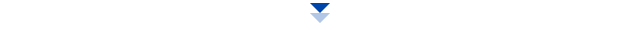Seaborn可以使用一行代码绘制数据集的线性回归拟合图表。本期小哥以Seaborn中的Tips数据集讲解如何绘制。Seaborn中可使用lmplot()和regplot()两种函数绘制线性回归拟合图表。其中，lmplot()是regplot()和FacetGrid的结合体。所以掌握了regplot()的用法就掌握了另外一种。为了方便展示，本次分析小哥在Jupyter Notebook中进行。参考链接：http://seaborn.pydata.org/generated/seaborn.lmplot.html#seaborn.lmplothttp://seaborn.pydata.org/generated/seaborn.regplot.html#seaborn.regplot1. Tips数据集首先导入数据集，观察一下数据集的基本情况。import seaborn as sns
sns.set(font='SimHei', font_scale=1.3) #设置字体大小
sns.set_style("whitegrid") # 设置绘图style
# 获取数据集
df = sns.load_dataset("tips")
# 查看数据前几行
df.head()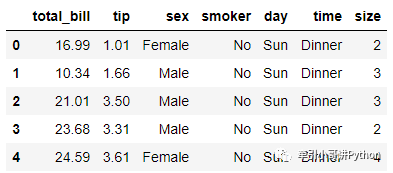2. regplot()绘制线性回归拟合# 绘制total_bill和tip的拟合图表：
sns.regplot(x="total_bill", y="tip", data=df)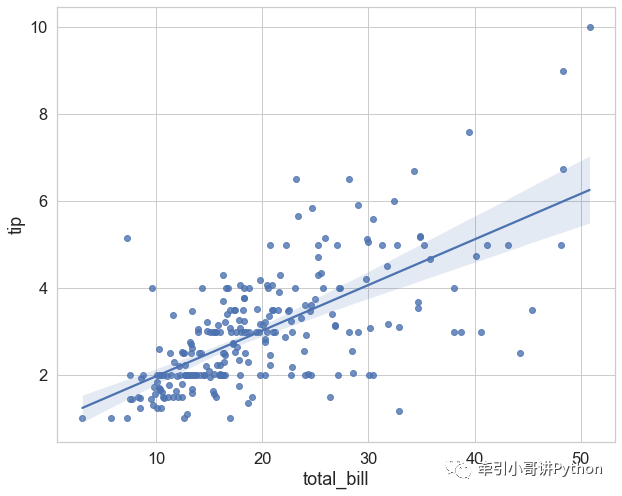从结果我们可以看到，regplot()的拟合带有置信度（默认95%）。可以使用ci进行调整，调整范围为：[0, 100]整数 or None。# 关闭置信区间
sns.regplot(x="total_bill", y="tip", ci=None, data=df)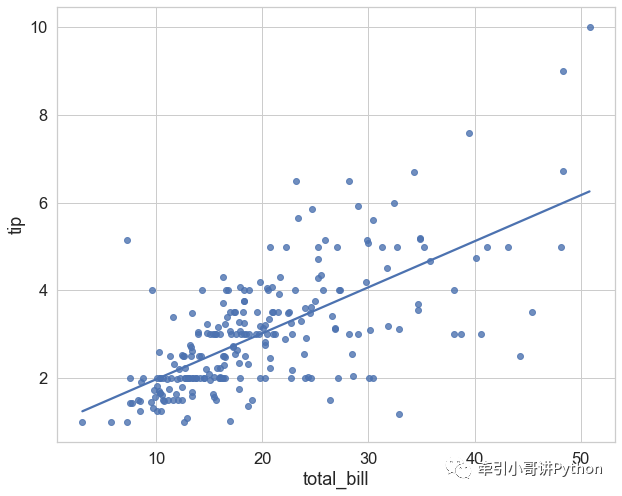# 绘制多个变量的拟合，通过 hue 映射不同的颜色
sns.lmplot(x="total_bill", y="tip", hue="smoker", data=df)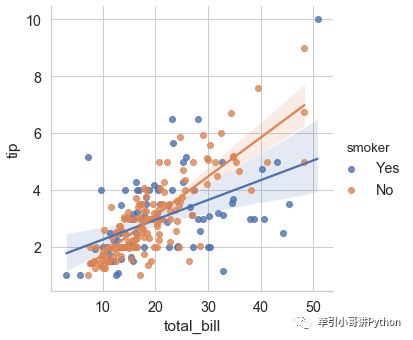# 绘制多个变量的拟合，通过 hue 映射不同的颜色。
# 定义不同的标志符号，设置调色盘为‘Set1’
sns.lmplot(x="total_bill", y="tip", hue="smoker", data=df,
markers=["o", "x"], palette="Set1")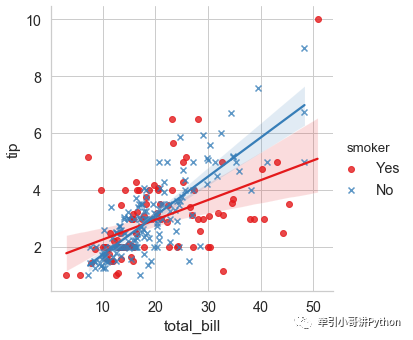3. lmplot()绘制线性回归拟合Seaborn有一个非常强大的功能：FacetGrid。这种功能可自动根据变量种类形成分类绘制子图映射。而lmplot()中就包含这种功能。首先讲解一下思路，在上一节中绘制了这样一个图表：在这个图表中，根据顾客是否吸烟（smoker）绘制了两条回归曲线。那么，如果想要单独绘制这两条曲线在一幅图中，并且有标记呢？这时就可以用到lmplot()，该函数中主要使用col，row，hue三个参数控制子图的行列和颜色映射，话不多说，直接看代码。sns.lmplot(x="total_bill", y="tip", col="smoker", hue='smoker', markers=["o", "x"], data=df)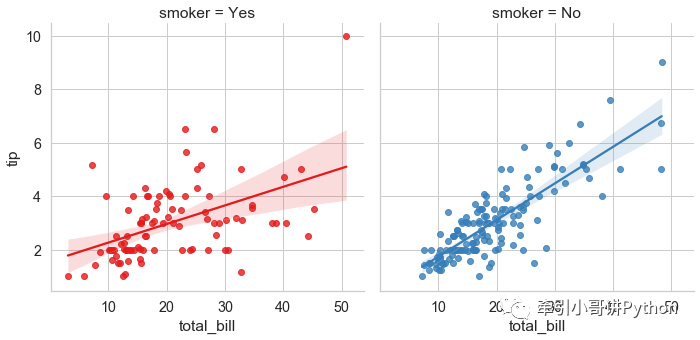为了展示这种绘图方法的优越性，再以day这一列进行分类：# 使用 col 参数控制
sns.lmplot(x="total_bill", y="tip", hue="day", col="day", palette="Set1", data=df)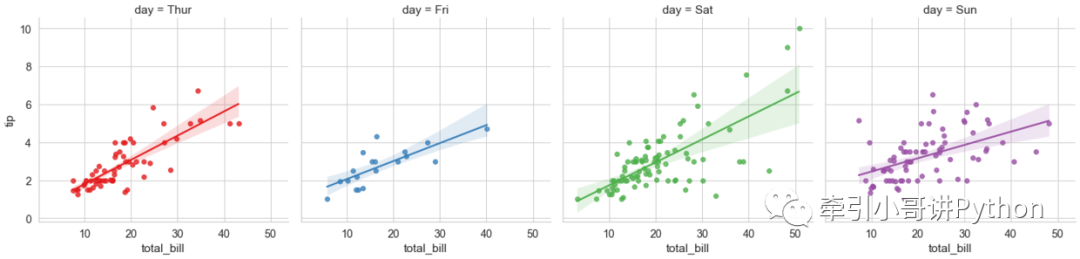# 使用 row 参数控制
sns.lmplot(x="total_bill", y="tip", hue="day", row="day", palette="Set1", data=df)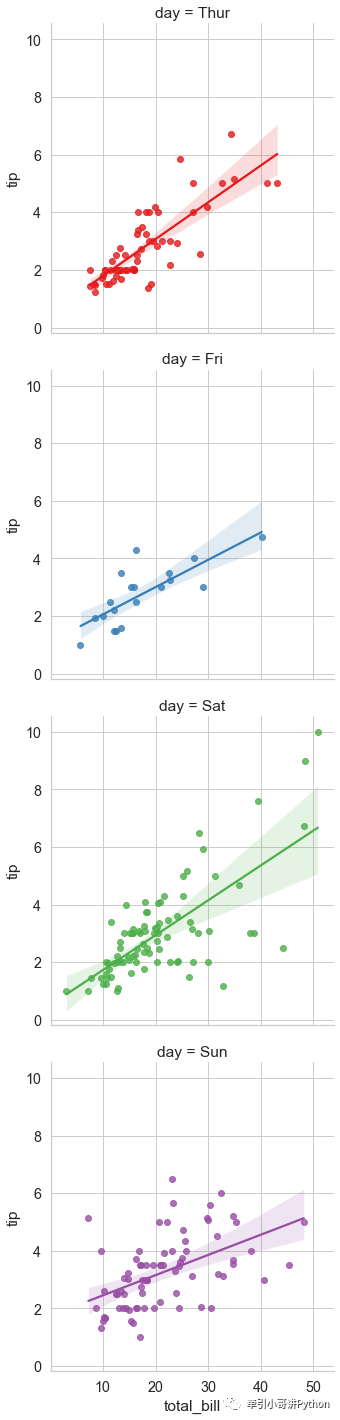# 绘制2x2的子图布局，使用 col_wrap 参数
sns.lmplot(x="total_bill", y="tip", hue="day",
col="day", col_wrap=2, palette="Set1", data=df)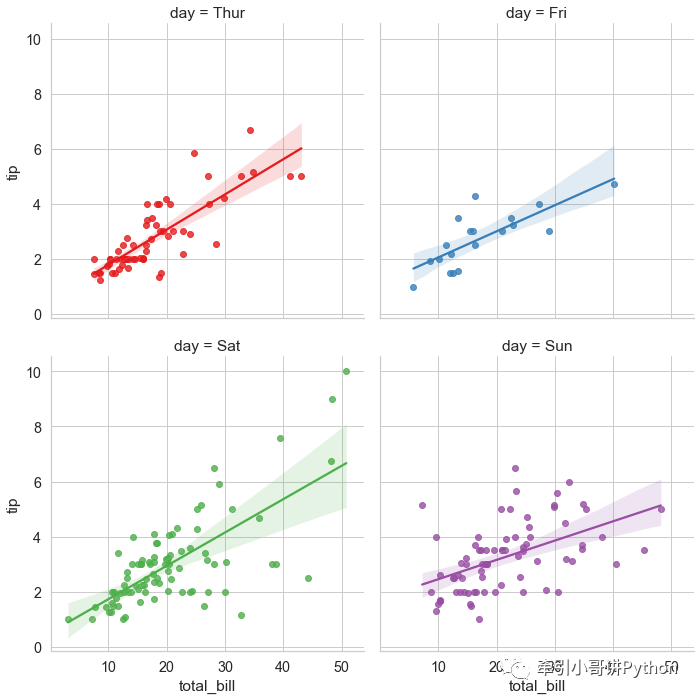文末福利各位猿们，还在为记不住API发愁吗，哈哈哈，最近发现了国外大师整理了一份Python代码速查表和Pycharm快捷键sheet，火爆国外，这里分享给大家。这个是一份Python代码速查表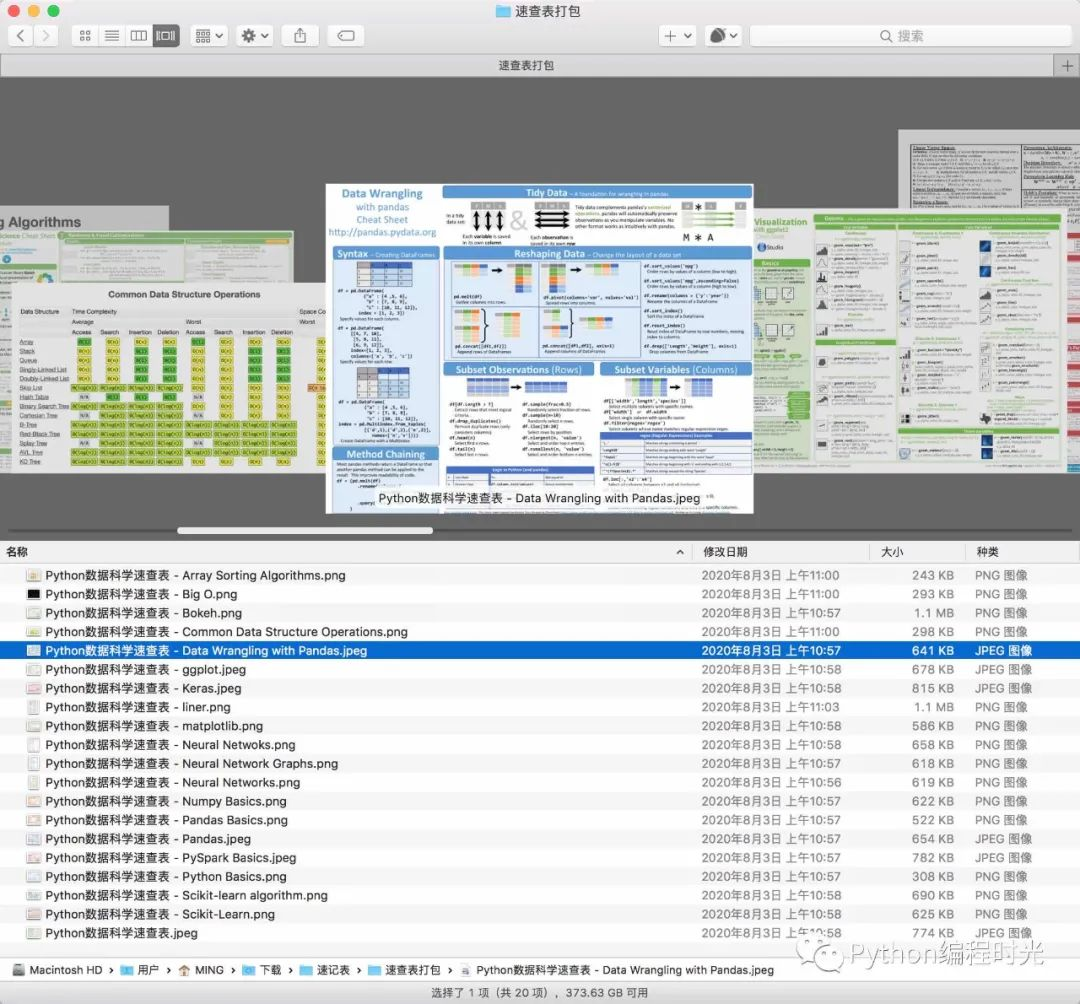下面的宝藏图片是2张(windows && Mac)高清的PyCharm快捷键一览图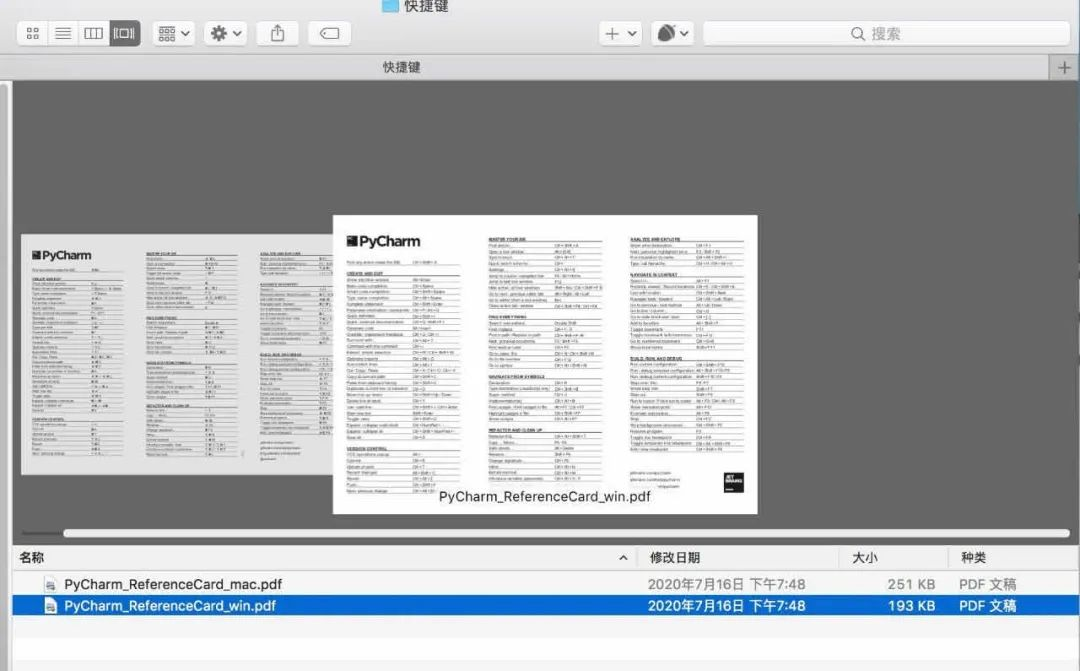怎样获取呢？可以添加我们的AI派团队的程序媛姐姐一定要备注【高清图】哦????????????????????➕我们的程序媛小姐姐微信要记得备注【高清图】哦来都来了，喜欢的话就请分享、点赞、在看三连再走吧~~~展开全文python css 数据可视化 html 支持向量机
• .WORD 版本 Matlab 线性回归(拟合)对于多元线性回归模型：e x x y p p ++++=βββ 110设变量12,,,p x x x y 的n 组观测值为12(,,,)1,2,,i i ip i x x x y i n =．记 ⎪⎪⎪⎪⎪⎭⎫ ⎝⎛=np n n p p x x x x x x x ...
.WORD 版本 Matlab 线性回归(拟合)对于多元线性回归模型：e x x y p p ++++=βββ 110设变量12,,,p x x x y 的n 组观测值为12(,,,)1,2,,i i ip i x x x y i n =．记 ⎪⎪⎪⎪⎪⎭⎫     ⎝⎛=np n n p p x x x x x x x x x x         212222111211111，⎪⎪⎪⎪⎪⎭⎫     ⎝⎛=n y y y y  21，则⎪⎪⎪⎪⎪⎭⎫     ⎝⎛=p ββββ 10 的估计值为 y x x x b ')'(ˆ1-==β(11.2) 在Matlab 中，用regress 函数进行多元线性回归分析，应用方法如下：语法：b = regress(y, x)[b, bint, r, rint, stats] = regress(y, x)[b, bint, r, rint, stats] = regress(y, x, alpha)b = regress(y, x)，得到的1+p 维列向量b 即为(11.2)式给出的回归系数β的估计值．[b, bint, r, rint, stats]=regress(y, x) 给出回归系数β的估计值b ，β的95％置信区间((1)2p +⨯向量)bint ，残差r 以及每个残差的95％置信区间(2⨯n 向量)rint ；向量stats 给出回归的R 2统计量和F 以及临界概率p 的值．如果i β的置信区间(bint 的第1i +行)不包含0，则在显著水平为α时拒绝0i β=的假设，认为变量i x 是显著的．[b, bint, r, rint, stats]=regress(y, x, alpha) 给出了bint 和rint 的100(1-alpha)%的置信区间．三次样条插值函数的MATLAB 程序
展开全文• # -*- coding: utf-8 -*- """ ...线性回归拟合直线 @author: Han """ from keras.models import Sequential from keras.layers import Dense import numpy as np# 创建一个等差数列 trX = np.linspa
# -*- coding: utf-8 -*-
"""
Created on Sat Aug 26 18:25:28 2017
线性回归拟合直线
@author: Han
"""
from keras.models import Sequential
from keras.layers import Dense
import numpy as np
import matplotlib.pyplot as plt

# 创建一个等差数列
trX = np.linspace(-1 , 1 , 100)
trY = 3 * trX + np.random.randn(*trX.shape) * 0.33

# 绘制数据
plt.scatter(trX , trY)
plt.show()

# 分训练集和测试集
X_train  , y_train = trX[:80] , trY[:80]
X_test , y_test = trX[80:] , trY[80:]

model = Sequential()
model.add(Dense(input_dim = 1 , output_dim = 1 , init = 'uniform' , activation='linear'))

# 选择损失函数和优化方法
model.compile(optimizer='sgd' , loss='mse')
model.fit(X_train , y_train , epochs=200 , verbose=1)

# 获取参数
W , b = model.layers.get_weights()
print('Linear regression model is initialized with weights w: %.2f, b: %.2f' % (W, b))

# 测试
Y_pred = model.predict(X_test)
plt.scatter(X_test , y_test)
plt.plot(X_test , Y_pred)
plt.show()

# 保存权重
model.save_weights('my_model.h5')
model.load_weights('my_model.h5')

`
展开全文• Prism使得将模型拟合到数据非常容易，本篇的内容比较简单直接，手把手教大家如何使用非线性回归拟合模型——如何用Prism拟合模型。 步骤1：输入数据 创建一个XY工作台，并输入数据。如果在每个X值上均有重复的Y...
• 关于多元线性回归的拟合以及出图，其大致方法与简单线性回归类似，方法详细介绍请参照我的博客Python关于如何进行简单线性回归拟合以及出图 这里直接列出代码不再做介绍 # -*- coding: utf-8 -*- import numpy as ...PYTHON
• Python进阶量化交易场外篇2——线性回归拟合股价沉浮这里只要给大家介绍下搭建环境中可能会遇到的问题，如果有同学是用mac系统开发的，在MAC中调试matplotlib时中文显示框框解决方法：1、下载simhei.ttf字体库拷贝至...
•数据分析 r语言
• 背景资料随着海拔高度的上升，温度越来越低，经过气象专家的...解决思路采用sklearn库中的LinearRegression线性回归类进行拟合。代码# 导入所需的模块 import numpy as np import matplotlib.pyplot as plt from skl...
• 1.线性回归:regress 顾名思义，就是一元多元方程的的拟合，y=c1*x1+c2*x2....或者y=c1*x1^2+c2*x2^2+c3*x1*x2....等等形式[b,BINT] = regress(Y,X)[b,BINT,R] = regress(Y,X)[b,BINT,R,RINT] = regress(Y,X)[b,BINT,...
• matlab多元线性回归拟合 a=[320 320 160 710 320 320 320];f=[0.18 0.18 0.18 0.18 0.09 0.36 0.18];v=[2.3 1.7 1.7 1.7 1.7 1.7 1];F=[38.8 29.23 26.53 25.28 19.53 35.72 16.75];模型：lnF=lnA+Blna+Clnf+Dlnv...
• 线性拟合：对于y=a*x+b的形式a=(N*ΣXi*Yi-ΣXi*ΣYi)/(N*ΣXi*Xi-(ΣXi)2)b=((ΣXi*Xi)*(ΣYi)-(ΣXi)*(ΣXi*Yi))/(N*ΣXi*Xi-(ΣXi)2)利用Matlab自带函数可实现：拟合函数：pn=polyfit(x,y,n) 返回pn系数向量，降...
• # 建立线性回归模型 regr = linear_model.LinearRegression() # 拟合 regr.fit(X, Z) # 不难得到平面的系数、截距 a, b = regr.coef_, regr.intercept_ # 给出待预测的一个特征 x = np.array([[5.8, 78.3]]) # ...
• 原标题：使用线性回归拟合平面最佳直线及预测之Python+sklearn实现本文代码采用sklearn扩展库实现，使用线性回归算法解决下面的问题：根据平面上已知3个点的坐标，拟合最佳直线斜率k和截距b，然后根据拟合的结果对给...
• Python进阶量化交易场外篇2——线性回归拟合股价沉浮这里只要给大家介绍下搭建环境中可能会遇到的问题，如果有同学是用mac系统开发的，在MAC中调试matplotlib时中文显示框框解决方法：1、下载simhei.ttf字体库拷贝至...
• 上一篇文章使用最小二乘法来拟合直线，有一个重要的缺点就是仅考虑了因变量 存在误差的情况，但是很多情况下，原始点的横纵坐标都会有误差存在。本文使用正交回归的方法，解决了最小二乘的两个缺点：同时考虑了横纵...
•     简单的讲，有一堆数据，需要简单的拟合出一条直线，如： 我们知道其表达式是： y=wx+b y=wx+b y=wx+b 那么，我们根据损失函数的思路，可以很容易得出其损失函数： Loss=12m∑i=0N(y^−y)2=12m∑i=0N(wx+b−...
• 2.函数返回值及意义b 回归系数，是一个一维向量，第一个是回归方程的常数b0bint 回归系数的置信区间，是一个2列的矩阵r 残差rint 残差置信区间，是一个2列的矩阵stats用于检验回归模型的统计量，有4...
• 拟合了Y关于X的线性模型之后，我们不但想知道这种线性关系是否真的存在，还想度量模型对数据的拟合效果。拟合效果可以采用下面的方法之一进行度量，这些方法有很高的关联性。 1.Y对X的散点图、Y对Y的散点图 Y...机器学习 人工智能
• 线性回归拟合正弦函数 %matplotlib inline import matplotlib.pyplot as plt import numpy as np n_dots = 200 X = np.linspace(-2 * np.pi, 2 * np.pi, n_dots) Y = np.sin(X) + 0.2 * np.random.rand(n_d...
• Python进阶量化交易场外篇2——线性回归拟合股价沉浮这里只要给大家介绍下搭建环境中可能会遇到的问题，如果有同学是用mac系统开发的，在MAC中调试matplotlib时中文显示框框解决方法：1、下载simhei.ttf字体库拷贝至...
• order--默认为1,即拟合直线,可设置为大于1的整数,即拟合多项式的次数;logx--默认为False,设置为True则回归分析时自变量取对数;x_jitter--取值为小数,在绘制离散变量回归曲线时,给数据横坐标增加噪声;y_jitter--取值...
• 机器学习:形如抛物线的散点图在python和R中的非线性回归拟合方法对于样本数据的散点图形如函数y=ax2+bx+c的图像的数据， 在中的拟合过程为：##最小二乘法import numpy as npimport scipy as spimport matplotlib....
• R方由协变量X解释的结果Y的变化比例通常被描述为拟合优度的度量。这当然看起来非常合理，因为R平方测量观察到的Y值与模型的预测（拟合）值的接近程度。 然而，要记住的重要一点是，R平方不会向我们提供有关我们...R方...